# Design Data in Ultrasonic Transducers for Use in Air

High-intensity ultrasonic sound levels can be easily generated under water, and they can also be easily transmitted over ranges of several miles. This accounts for various advancements in the use of underwater sound for a variety of applications.

The high acoustic impedance of the liquid, which needs relatively small amplitudes of vibration in the medium to produce high acoustic power levels, makes it relatively easy to generate high-intensity sound energy under water.

The attenuation of ultrasonic sound in water is considerably less than the attenuation of sound of the same frequency in air, making it viable to transmit high-intensity underwater sound across extremely large distances.

The low acoustic impedance of the medium, which needs relatively large amplitudes of vibration, can considerably limit the generation of high-intensity sound fields in air. However, there are practical limits in the highest amplitudes of vibration, which may be produced by ultrasonic transducers.

Two of the most major limitations are imposed by:

• the maximum stress that can be safely allowed in the vibrating element without reaching fatigue failure
• the harmonic distortion produced in the air when the peak acoustic pressure becomes an appreciable fraction of atmospheric pressure

Different types of ultrasonic transducers are available that are suitable for use in air. This article presents some fundamental design data to provide a quantitative understanding of the numerous relationships between some of the fundamental parameters that control the generation and transmission of ultrasonic sound in air.

## Design Data

This section presents some useful fundamental information regarding the quantitative relationships between frequency, piston displacements, sound pressure level, acoustic power, attenuation, beam patterns, etc. to enable the hypothetical analysis of a transducer design to generate ultrasonic power of a specified intensity.

### Acoustic Power vs. Piston Displacement and Frequency

Setting a plane surface into vibration with uniform amplitude and phase across its entire area provides a true piston, the air’s radiation resistance against which the piston is vibrating becomes constant for the frequency region where the diameter of the piston is greater than 13 wavelength of the sound.

In the cgs system, the extent of this impedance for normal atmospheric conditions is roughly equal to 42 acoustic ohms per square centimeter of piston area. A vibrating piston that generates the acoustic power under such conditions  is given by: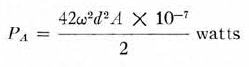(1)

Where

ω = 2πf
f = frequency in c/s
d = peak displacement of piston in cm
A = area of piston in cm2

Figure 1 shows a design chart derived from (1), displaying the relationship between the acoustic power radiated from the surface at various frequencies and the peak displacement of a vibrating surface. For 0.001” peak displacement, it can be seen that only a few watts per square inch can be produced at the lower ultrasonic frequencies.Figure 1. Relationship between peak displacement of a vibrating surface and the acoustic power generated at various frequencies. (Surface diameter is greater than 13 wavelength.)

### Acoustic Power vs. Sound Pressure

The sound pressure at a distance of one foot from an acoustic source is shown in Figure 2. This sound pressure is expressed in microbars as a function of the acoustic power in watts being radiated by the source. The chart shown in Figure 2 assumes that the source is radiating uniformly into a hemispherical space, neglecting attenuation due to loss of viscosity in the air.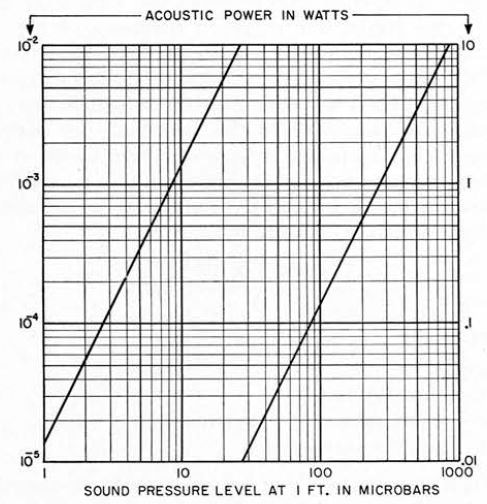Figure 2. Sound-pressure level at one foot vs. acoustic power output from an omnidirectional source radiating into hemispherical space.

### Increase in Sound Pressure Along Normal Axis of a Large Piston

For conditions where the vibrating source is a circular piston - whose diameter is larger than that of the wavelength of sound being generated - the sound radiation is limited within a tiny conical beam, which leads to a relative increase in the sound-pressure level along the standard axis of the vibrating piston.

The magnitude of the increase in the axial sound pressure is shown in Figure 3. This axial sound pressure results from the concentration of the sound as a function of the ratio of the piston diameter over the wavelength of sound being radiated.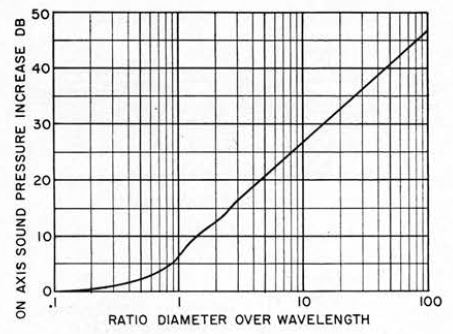Figure 3. Increase in sound-pressure level along the normal axis of a piston generating constant acoustic power as a function of the ratio of piston diameter to wavelength of sound.

### Beam Pattern vs. Piston Diameter

Figure 4 displays a series of curves that could be used to measure the shape of the main beam into which the sound generated from a large vibrating piston is confined. The beam angles shown by the four curves is the point at which response is down 3, 6, 10, and 20 dB as a function of the diameter of the piston over the wavelength of sound being radiated.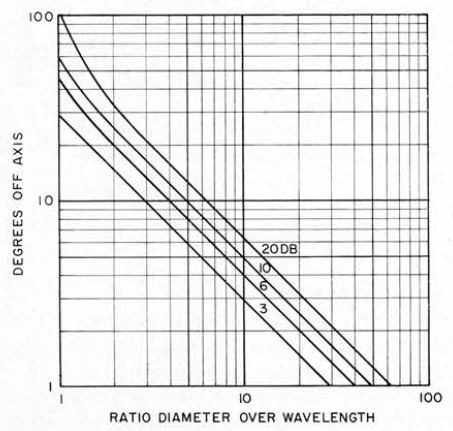Figure 4. Directional radiation pattern from a vibrating circular piston showing the degrees off the normal axis at which the attenuation is 3, 6, 10, and 20 dB as a function of piston diameter over wavelength.

### Attenuation of Ultrasonic Sound in Air

The relatively high attenuation resulting from the loss of viscosity caused by the molecular vibrations considerably limits the transmission of ultrasonic frequencies in air. Knudsen  investigated the absorption of sound in air and discovered that in the presence of water vapor in the air, the absorption increases and becomes greater than the Stokes  classical absorption, which applies for dry air.

According to Knudsen, the maximum attenuation occurs in the area of about 20% relative humidity. Sivian , conducted additional investigations on the absorption of high frequency sounds in the atmosphere.

Figure 5 shows his reported data as curve A, which represents the experimental absorption as a function of frequency for average atmospheric conditions corresponding to 37% relative humidity and 75°F at standard atmospheric pressure.

The attenuation calculated from the Stokes classical theory is curve B. As measured by Sivian, the dry-air attenuation data was about 50% higher than the values indicated by curve B.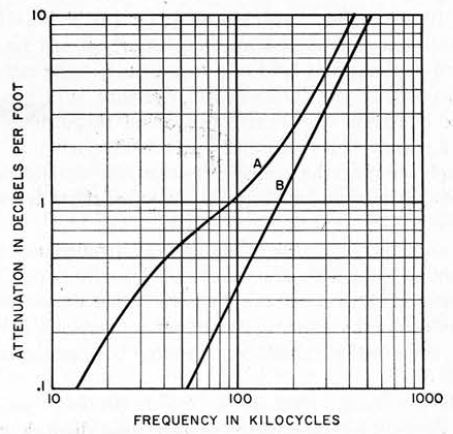Figure 5. Absorption of sound in as a function of frequency. Curve A is the average of experimental data for average atmospheric conditions 75°F and 37 percent relative humidity. Curve 71 is the theoretical attenuation for dry air from classical theory.

### Acoustic Power Output of Nonpiston Sources

For sirens, modulated air-flow loudspeakers, and other such nonpiston-type sound sources, the acoustic power produced by the source  is given by: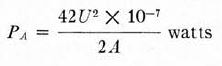(2)

Where

U = peak volume velocity of air through port opening in cm3/s.
U = 2πfX
X = peak volume displacement though opening in cm2
f = frequency in c/s
A = area of opening in cm2

On initial analysis, it may appear that (2) is incorrect, because it states that for a fixed volume velocity of air moving through an opening, there is an increase in acoustic power as the opening is made smaller.

This is how it should be, because as the opening area reduces, the velocity of the fluid flowing via the opening increases in inverse proportion. Given that the acoustic power radiated for each unit area is proportional to the square of the fluid velocity, the relationship in (2) will follow.

However, there are two limitations that prevent it from merely reducing, without limit, the port opening of a modulated air-flow transducer to amplify the power created for a continuous fluid displacement. Linear dimensions should be in the discharge opening and these should not become smaller than 13 wavelength of the sound being produced so that the radiation resistance per unit area of the opening remains constant.

In addition, as the area decreases and the acoustic power density increases with a corresponding increase in sound pressure, the highest sound pressure produced should remain a small percentage of the atmospheric pressure, or distortion will be introduced due to nonlinearity in the medium.

The generation of extraneous harmonic frequencies in air due to the presence of powerful sound waves was studied by Thuras, Jenkins, and O'Neil , who discovered that the second harmonic distortion, which is created in the region of high-intensity sound pressure, is directly proportional to the magnitude and frequency of sound pressure.

During every cycle of sound pressure oscillation, if equal negative and positive increments of pressure are impressed on a mass of air, then the changes in mass volume will not be equal. The changes in volume for the positive pressure will be less compared to the change in volume for the equal negative pressure.

When the ac pressure becomes an appreciable part of atmospheric pressure, the harmonic generation from this nonlinear condition in the air becomes extremely high.

For a peak sound pressure of the order of 110 atmosphere, which is equal to +171 dB vs. 0.0002 µbar, a second harmonic distortion will be produced in the sound field of a magnitude of the order of 10% of the fundamental pressure within a distance of approximately a single wavelength along the propagation direction.

This assumes that the frequency is high enough that the sound pressure has not significantly reduced over a distance of a single wavelength from the source.

If an arbitrary value of approximately 110 atmosphere is assumed as the peak value of sound pressure that has to be produced with the highest acceptable distortion, then the acoustic power per square inch of radiating area of the transducer will be restricted to about 77 W/in2.

Figure 6 shows a series of curves derived from (2), indicating the association between acoustic-power output, fluid displacement, and frequency for different areas of openings in a modulated air-flow loudspeaker. The dotted line drawn across the chart represents the upper limit of acoustic power which is acceptable for numerous radiating areas to maintain the peak sound pressure at the source under 110 atmosphere.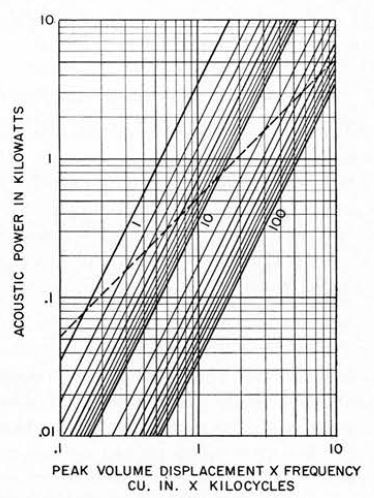Figure 6. Relationship between peak volume displacement, frequency, and acoustic power output for modulated air-flow transducers. Area of port opening in square inches is indicated on curves. Dotted line represents upper limit of acoustic power at which peak sound pressure near source becomes equal to 110 atmosphere.

References

 H. F. Olson and F. Massa, Applied Acoustics, 2nd ed. Philadelphia, Pa.: Blakiston, 1939, sect. 1.7.

 V. O. Knudsen, J. Acoust. Soc. Am., vol. 5, p. 112, October 1933.

 G. G. Stokes, Trans. Am. Phil. Soc., vol. 8, p. 287, 1845.

 L. J. Sivian, J. Acoust. Soc. Am., vol. 19, p. 914, September 1947.

 H. F. Olson and F. Massa, ob. cit., ch. 2.

 A. L. Thuras, R. T. Jenkins, and H. T. O’.N'eil, J. Acoust. Soc. Am., vol. 6, p. 173, January 1935.

 J. Hartmann, J. Sei. Instr., vol. 16, p. 141, May 1939.

 C. H. Allen and I. Rudnik, J. Acoust. Soc. Am., vol. 19, p. 857, September 1947.

 P. M. Morse, Vibration and Sound. New York: McGraw-Hill, 1936, p. 173.

[9a] G. Rasmus.sen, 1962 Proc. 4th Internat'l Congress on Acoustics, paper N51; reissued in Brüel and Kjaer Technical Review No. 1, p. 3, 1963.

 W. K. Kuhl, G. R. Schodder, and F.-K. Schröder, Acoustica, vol. 4. p. 519, 1954.

[11 W. M. Wright, 1962 IRE Nat’l Conv. Rec., vol. 10, pt. 6, p. 95.

 F. Massa, J. Acoust. Soc. Am., vol. 17, p. 29, July 1945.

 —, J. Acoust. Soc. Am., vol. 20, p. 451, July 1948.

 —, Electronics, p. 128, May 1960.

 —, 1960 IRE Nat’l Conv. Rec., vol. 8, pt. 6, p. 243.This information has been sourced, reviewed and adapted from materials provided by Massa Products Corp.

## Citations

• APA

Massa Products Corp.. (2019, July 23). Design Data in Ultrasonic Transducers for Use in Air. AZoSensors. Retrieved on June 03, 2020 from https://www.azosensors.com/article.aspx?ArticleID=709.

• MLA

Massa Products Corp.. "Design Data in Ultrasonic Transducers for Use in Air". AZoSensors. 03 June 2020. <https://www.azosensors.com/article.aspx?ArticleID=709>.

• Chicago

Massa Products Corp.. "Design Data in Ultrasonic Transducers for Use in Air". AZoSensors. https://www.azosensors.com/article.aspx?ArticleID=709. (accessed June 03, 2020).

• Harvard

Massa Products Corp.. 2019. Design Data in Ultrasonic Transducers for Use in Air. AZoSensors, viewed 03 June 2020, https://www.azosensors.com/article.aspx?ArticleID=709.